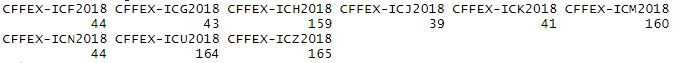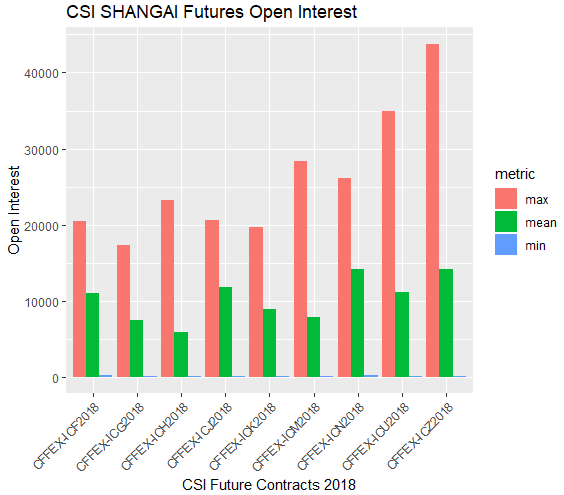# Exploring Open Interest for Futures Contracts with R

In this article we will explore in greater depth the Open Interest variable of the futures contracts price data and see in which months of the year this variable tends to increase for a particular contract. For this purpose, we have gathered contracts futures data from CSI 500 Shanghai Index

Our task will be to load all CSV files from a specific folder in R environment, read each file as a data frame and returns a list structure where each item is the contract code and the values of the list are the Open Interest values on each trading day. We will call this function openInterest and the directory parameter is the folder that stores all the csv files with the individual contract information.

With this structure we can obtain the min, max and mean Open Interest by contract for a specific year.

openInterest <- function(directory){
data <- c(list.files(directory))
files <- paste(directory,data,sep="/")
contractsNumber <- length(files)
OI <- list()
# Loop over all contracts in the directory folder
for (contract in 1:contractsNumber){
openInterest <- c()
# Retrieve the open Interest column that is in the 9 position
openInterest <- contractData[,9]
OI[[contract]] <- openInterest
}
names(OI) <- gsub('.csv','',list.files(directory))
return(OI)
}

OI <- openInterest("C:/Users/Nicolas/Documents/Shanghai CSI")

# The contracts have different periods. Using the sapply command with the OI list
# we can view the number of trading days for each contract.

contracts_Length <- sapply(OI,length)

# contracts_Length has the following values:# Remove the first Open Interest value in all contracts which is zero. We want to #know the min value of Open Interest for each contract, but should remove 0 that is #when the contract starts. This function will keep only values from each item of the #OI list that are different from zero.

newOIList <- lapply(OI, function(i) Filter(function(x) any(x != 0), i))

# Calculate mean, max and min for each item of the newOIList. This would return
# all maxs,means and mins Open Interest values for each particular contract.

mean<- sapply(newOIList,mean,na.rm=TRUE)
max <- sapply(newOIList,max,na.rm=TRUE)
min <- sapply(newOIList,min,na.rm=TRUE)

# Make a data frame with cbind command that combine the three lists by columns

OI_metrics <- data.frame(cbind(max,min,mean))

# Create a column with the contracts code and reset the index by assign NULL to r
# rownames.

OI_metrics$Contract <- row.names(OI_metrics) rownames(OI_metrics) <- NULL #OI_metrics is a dataframe with wide format with the following values: max min means Contract 1 20457 228 11054 CFFEX-ICF2018 2 17308 165 7451 CFFEX-ICG2018 3 23228 95 5825 CFFEX-ICH2018 4 20545 67 11786 CFFEX-ICJ2018 5 19696 81 8848 CFFEX-ICK2018 6 28363 162 7798 CFFEX-ICM2018 7 26187 232 14225 CFFEX-ICN2018 8 34943 116 11126 CFFEX-ICU2018 9 43776 131 14167 CFFEX-ICZ2018 # Next step is to transform the data from wide to long in order to make a grouped # barplot and show the metrics values by contract. The OI_metrics has a wide format #now, because each contract is in a unique row. In order to make a barplot we need #to change the format to long format. The gather command from dplyr package #transform the shape of the data from wide to long. The purpose of this is to get #tidy data for their analysis. long_OI_metrics <- gather(OI_metrics, metric, value ,c('max','min','mean')) # Use order command with Contract column to sort the dataframe by contract name OI_metricsByContracts <- long_OI_metrics[order(long_OI_metrics$Contract),]

# The OI_metricsByContracts is a dataframe with long format that allow us to create a barplot by year.

Contract     metric value
1  CFFEX-ICF2018    max 20457
10 CFFEX-ICF2018    min   228
19 CFFEX-ICF2018  means 11054
2  CFFEX-ICG2018    max 17308
11 CFFEX-ICG2018    min   165
20 CFFEX-ICG2018  means  7451
3  CFFEX-ICH2018    max 23228
12 CFFEX-ICH2018    min    95
21 CFFEX-ICH2018  means  5825
4  CFFEX-ICJ2018    max 20545
13 CFFEX-ICJ2018    min    67
22 CFFEX-ICJ2018  means 11786
5  CFFEX-ICK2018    max 19696
14 CFFEX-ICK2018    min    81
23 CFFEX-ICK2018  means  8848
6  CFFEX-ICM2018    max 28363
15 CFFEX-ICM2018    min   162
24 CFFEX-ICM2018  means  7798
7  CFFEX-ICN2018    max 26187
16 CFFEX-ICN2018    min   232
25 CFFEX-ICN2018  means 14225
8  CFFEX-ICU2018    max 34943
17 CFFEX-ICU2018    min   116
26 CFFEX-ICU2018  means 11126
9  CFFEX-ICZ2018    max 43776
18 CFFEX-ICZ2018    min   131
27 CFFEX-ICZ2018  means 14167

# Finally we will use the ggplot2 package to plot the min, max and mean values of the gold mini future contract on 2017 from Indian Exchange

ggplot(OI_metricsByContract,aes(x=Contract,y=value,fill=metric)) +
geom_bar(stat="identity",position="dodge")+
xlab("Gold Mini Future Contracts 2017")+ylab("Open Interest") +
theme(axis.text.x = element_text(angle = 45, hjust = 1)) +ggtitle("CSI SHANGAI Futures Open Interest")


CSI 500 Shanghai Futures Open Interest MetricsFrom the barplot above, we can observe that the highest Open Interest  is in the CFFEX-ICZ2018 contract that is the Dec 2018 contract. Secondly, the CFFEX-ICU2018 future contract which is from Nov 2018 is the second highest traded contract in 2018. The contracts at the beginning of the year such as Jan, Feb, Mar, Apr and May have less trading activity than contracts at the end of the year.